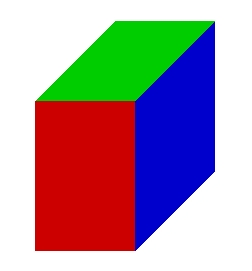# Dimensions 20553

The surface of the block is 558 cm², its dimensions are in the ratio 5: 3: 2.
Calculate the volume.

V =  810 cm3

### Step-by-step explanation:Did you find an error or inaccuracy? Feel free to write us. Thank you!

Tips for related online calculators
Do you have a linear equation or system of equations and looking for its solution? Or do you have a quadratic equation?